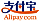Portada del libro de Classical Mechanics and Differential Geometry
Título del libro:

# Classical Mechanics and Differential Geometry

LAP LAMBERT Academic Publishing (2016-10-10 )apto para el cupón
ISBN-13:

### 978-3-659-96794-8

ISBN-10:
3659967947
EAN:
9783659967948
Idioma del libro:
Inglés
Notas y citas / Texto breve:
The book entitled ‘Classical Mechanics and Differential Geometry’ contains eight chapters. This book contains Variational Principle and Lagrange’s equations; Hamilton’s Principle, some techniques of calculus of variations, Derivation of Lagrange equations from Hamilton’s principle. Extension of principle to non holonomic systems. Conservation theorems and symmetry properties.Legendre transformations and the Hamilton equations of motion. Cyclic coordinates and conservation theorems. Routh’s procedure and oscillations about steady motion, The Hamiltonian formulation of relativistic mechanics, The Principle of least action. the equations of canonical transformation. Examples of canonical transformation. The simplistic approach to canonical transformations. Poisson brackets and other canonical invariants. Equations of motion. Infinitesimal canonical transformations and conservation theorems in the Poisson bracket formulation, the angular momentum, Poisson bracket relations, symmetry groups of mechanical systems. Liouville’s theorem. Definition of surface. Curves on a surface. Surfaces of revolution. Helicoids. Metric. Direction coefficients. Families of curves. Hilbert’s theorem.
Editorial:
LAP LAMBERT Academic Publishing
Sitio web:
https://www.lap-publishing.com/
Por (autor):
Namdeo Khobragade, Himanshu Roy
Número de páginas:
448
Publicado en:
2016-10-10
Stock:
Disponible
Categoría:
Matemáticas
Precio:
89.90 €
Palabras clave:
classical mechanics, differential geometry, Poisson bracket### Enlaces0 productos en el carrito
Editar carritoLOADING